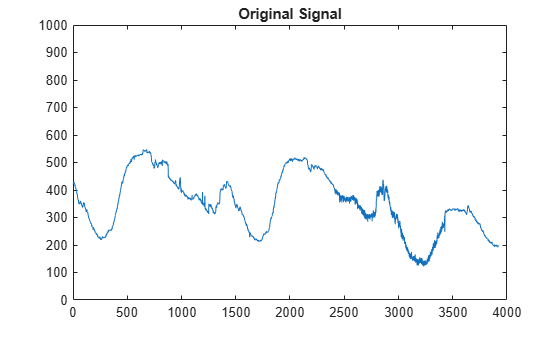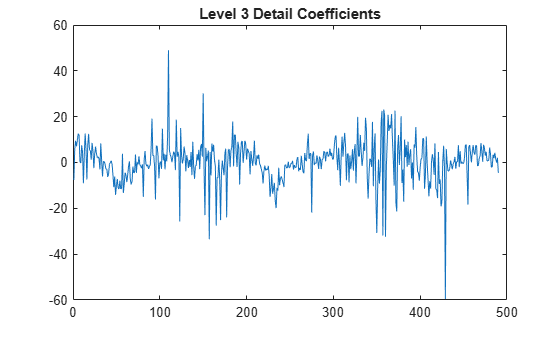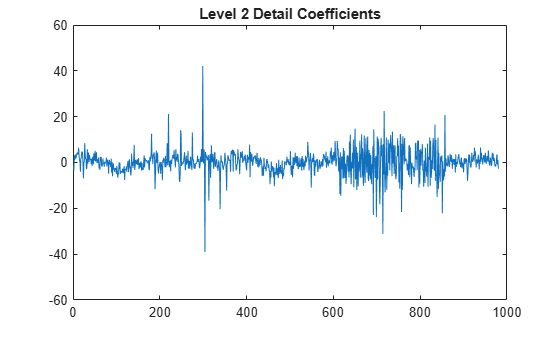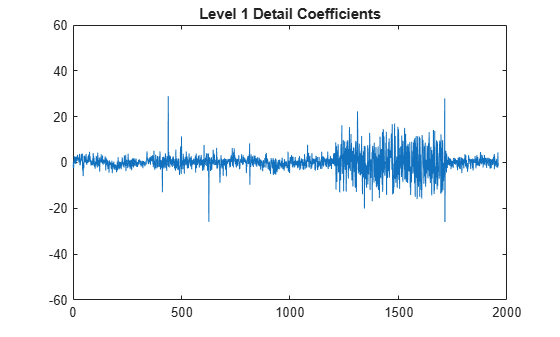Documentation

detcoef

1-D detail coefficients

Syntax

D = detcoef(C,L,N)
D = detcoef(C,L)

Description

detcoef is a one-dimensional wavelet analysis function.

D = detcoef(C,L,N) extracts the detail coefficients at level N from the wavelet decomposition structure [C,L]. See wavedec for more information on C and L.

Level N must be an integer such that 1 N NMAX where NMAX = length(L)-2.

D = detcoef(C,L) extracts the detail coefficients at last level NMAX.

If N is a vector of integers such that 1 ≤ N(j)NMAX:

• DCELL = detcoef(C,L,N,'cells') returns a cell array where DCELL{j} contains the coefficients of detail N(j).

• If length(N) > 1, DCELL = detcoef(C,L,N) is equivalent to DCELL = detcoef(C,L,N,'cells').

• DCELL = detcoef(C,L,'cells') is equivalent to DCELL = detcoef(C,L,[1:NMAX]).

• [D1, ... ,Dp] = detcoef(C,L,[N(1), ... ,N(p)]) extracts the details coefficients at levels [N(1), ... ,N(p)].

Examples

collapse all

This example shows how to obtain and plot the detail coefficients for an electrical current signal. This example uses zero-padding (see dwtmode).

Load the signal and select the first 3920 samples.

s = leleccum(1:3920);

Perform the decomposition at level 3 using db1. Extract the detail coefficients at levels 1, 2, and 3 from the decomposition structure.

[c,l] = wavedec(s,3,'db1');
[cd1,cd2,cd3] = detcoef(c,l,[1 2 3]);

Plot the original signal.

plot(s)
title('Original signal')
ylim([0 1000])Plot the level 3 detail coefficients.

plot(cd3)
title('Level 3 detail coefficients (cd3)')
ylim([-60 60])Plot the level 2 detail coefficients.

plot (cd2)
title('Level 2 detail coefficients (cd2)')
ylim([-60 60])Plot the level 1 detail coefficients.

plot (cd1)
title('Level 1 detail coefficients (cd1)')
ylim([-60 60])Extended Capabilities

C/C++ Code GenerationGenerate C and C++ code using MATLAB® Coder™.